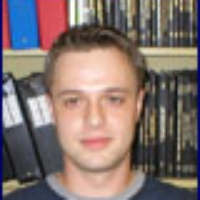# Matheus Grasselli, McMaster UniversityDepartment of Mathematics & Statistics Professor Hamilton, Ontario Office: (905) 525-9140 ext. 23417

## Bio/Research

My main research interests lie in the area of mathematical finance - the application of stochastic analysis and probability theory to the understanding and modelling of financial markets. Specific projects I am involved with include numerical and theoretical optimal portfolio selection and modell...

Click to Expand >>

## Bio/Research

My main research interests lie in the area of mathematical finance - the application of stochastic analysis and probability theory to the understanding and modelling of financial markets. Specific projects I am involved with include numerical and theoretical optimal portfolio selection and modelling of positive interest rates. I am also interested in classical and quantum information geometry. My work in this area ranges from the construction of infinite dimensional manifolds of probabilities to the applications of such geometric objects to models in fluid dynamics and quantum information.

Click to Shrink <<